efluids bicycle aerodynamics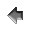Home Education FocusContact eFluidsChapters1. Introduction2. Adding Wind Speeds and Directions3. Characteristics of Fluids4. Pressure5. Continuity Equation6. Bernoulli's Equation7. Streamlines and Streamtubes8. Flows With Friction9. Transition and Turbulence10. Separation11. Drag of Blunt and Streamlined Bodies12. Drafting13. Golf Balls, Cricket Balls, and Tennis Balls14. Lift and Stall15. Useful LinkseFluids GalleriesGallery of ImagesGallery of VideosGallery of ExperimentsGallery of ProblemsGallery of Calculators## 6. Bernoulli's Equation

The Bernoulli equation states that,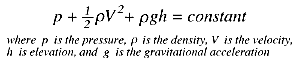where
• points 1 and 2 lie on a streamline,
• the fluid has constant density,
• the flow is steady, and
• there is no friction.
• Although these restrictions sound severe, the Bernoulli equation is very useful, partly because it is very simple to use and partly because it can give great insight into the balance between pressure, velocity and elevation.

How useful is Bernoulli's equation? How restrictive are the assumptions governing its use? Here we give some examples.

### 6.1 Pressure/velocity variation

Consider the steady, flow of a constant density fluid in a converging duct, without losses due to friction (figure 6.1). The flow therefore satisfies all the restrictions governing the use of Bernoulli's equation. Upstream and downstream of the contraction we make the one-dimensional assumption that the velocity is constant over the inlet and outlet areas and parallel.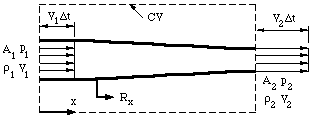Figure 6.1 One-dimensional duct showing control volume.

When streamlines are parallel the pressure is constant across them, except for hydrostatic head differences (if the pressure was higher in the middle of the duct, for example, we would expect the streamlines to diverge, and vice versa). If we ignore gravity, then the pressures over the inlet and outlet areas are constant. Along a streamline on the centerline, the Bernoulli equation and the one-dimensional continuity equation give, respectively,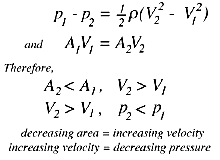These two observations provide an intuitive guide for analyzing fluid flows, even when the flow is not one-dimensional. For example, when fluid passes over a solid body, the streamlines get closer together, the flow velocity increases, and the pressure decreases. Airfoils are designed so that the flow over the top surface is faster than over the bottom surface, and therefore the average pressure over the top surface is less than the average pressure over the bottom surface, and a resultant force due to this pressure difference is produced. This is the source of lift on an airfoil. Lift is defined as the force acting on an airfoil due to its motion, in a direction normal to the direction of motion. Likewise, drag on an airfoil is defined as the force acting on an airfoil due to its motion, along the direction of motion.

An easy demonstration of the lift produced by an airstream requires a piece of notebook paper and two books of about equal thickness. Place the books four to five inches apart, and cover the gap with the paper. When you blow through the passage made by the books and the paper, what do you see? Why?

Two more examples:

#### Example 1

A table tennis ball placed in a vertical air jet becomes suspended in the jet, and it is very stable to small perturbations in any direction. Push the ball down, and it springs back to its equilibrium position; push it sideways, and it rapidly returns to its original position in the center of the jet. In the vertical direction, the weight of the ball is balanced by a force due to pressure differences: the pressure over the rear half of the sphere is lower than over the front half because of losses that occur in the wake (large eddies form in the wake that dissipate a lot of flow energy). To understand the balance of forces in the horizontal direction, you need to know that the jet has its maximum velocity in the center, and the velocity of the jet decreases towards its edges. The ball position is stable because if the ball moves sideways, its outer side moves into a region of lower velocity and higher pressure, whereas its inner side moves closer to the center where the velocity is higher and the pressure is lower. The differences in pressure tend to move the ball back towards the center.

#### Example 3

Suppose a ball is spinning clockwise as it travels through the air from left to right The forces acting on the spinning ball would be the same if it was placed in a stream of air moving from right to left, as shown in figure 6.2.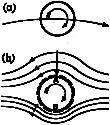Figure 6.2 Spinning ball in an airflow.

A thin layer of air (a boundary layer) is forced to spin with the ball because of viscous friction. At A the motion due to spin is opposite to that of the air stream, and therefore near A there is a region of low velocity where the pressure is close to atmospheric. At B, the direction of motion of the boundary layer is the same as that of the external air stream, and since the velocities add, the pressure in this region is below atmospheric. The ball experiences a force acting from A to B, causing its path to curve. If the spin was counterclockwise, the path would have the opposite curvature. The appearance of a side force on a spinning sphere or cylinder is called the Magnus effect, and it well known to all participants in ball sports, especially baseball, cricket and tennis players.

### 6.2 Stagnation pressure and dynamic pressure

Bernoulli's equation leads to some interesting conclusions regarding the variation of pressure along a streamline. Consider a steady flow impinging on a perpendicular plate (figure 6.3).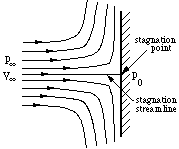Figure 6.3 Stagnation point flow.

There is one streamline that divides the flow in half: above this streamline all the flow goes over the plate, and below this streamline all the flow goes under the plate. Along this dividing streamline, the fluid moves towards the plate. Since the flow cannot pass through the plate, the fluid must come to rest at the point where it meets the plate. In other words, it ``stagnates.'' The fluid along the dividing, or ``stagnation streamline'' slows down and eventually comes to rest without deflection at the stagnation point.

Bernoulli's equation along the stagnation streamline giveswhere the point e is far upstream and point 0 is at the stagnation point. Since the velocity at the stagnation point is zero,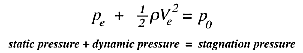The stagnation or total pressure, p_0, is the pressure measured at the point where the fluid comes to rest. It is the highest pressure found anywhere in the flowfield, and it occurs at the stagnation point. It is the sum of the static pressure (p_0), and the dynamic pressure measured far upstream. It is called the dynamic pressure because it arises from the motion of the fluid.

The dynamic pressure is not really a pressure at all: it is simply a convenient name for the quantity (half the density times the velocity squared), which represents the decrease in the pressure due to the velocity of the fluid.

We can also express the pressure anywhere in the flow in the form of a non-dimensional pressure coefficient C_p, whereAt the stagnation point C_p = 1, which is its maximum value. In the freestream, far from the plate, C_p = 0.

### 6.3 Pitot tube

One of the most immediate applications of Bernoulli's equation is in the measurement of velocity with a Pitot-tube. The Pitot tube (named after the French scientist Pitot) is one of the simplest and most useful instruments ever devised. It simply consists of a tube bent at right angles (figure 6.4).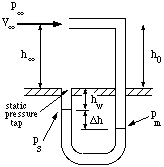Figure 6.4 Pitot tube in a wind tunnel.

By pointing the tube directly upstream into the flow and measuring the difference between the pressure sensed by the Pitot tube and the pressure of the surrounding air flow, it can give a very accurate measure of the velocity. In fact, it is probably the most accurate method available for measuring flow velocity on a routine basis, and accuracies better than 1% are easily possible. Bernoulli's equation along the streamline that begins far upstream of the tube and comes to rest in the mouth of the Pitot tube shows the Pitot tube measures the stagnation pressure in the flow. Therefore, to find the velocity V_e, we need to know the density of air, and the pressure difference (p_0 - p_e). The density can be found from standard tables if the temperature and the pressure are known. The pressure difference is usually found indirectly by using a ``static pressure tapping'' located on the wall of the wind tunnel, or on the surface of the model.© Copyright on the images is held by the contributors. Apart from Fair Use, permission must be sought for any other purpose.